# Can One Hear the Shape of a Drum? Part 2, Eigenfunctions

This is the second part of a series of posts about Marc Kac's 1966 paper in the American Mathematical Monthly . This part is devoted to contour plots of the eigenfunctions.

### Contents

#### Eigenfunctions

I described the isospectral drums in part 1. Contour plots of the eigenfunctions are beautiful. Here are the first twenty. The detail increases as the frequency increases. Notice the triangles created by the ninth eigenfunction. These triangles play a central role in the next part of this article.
   % Vertices
drum1 = [0 0 2 2 3 2 1 1 0
0 1 3 2 2 1 1 0 0];
drum2 = [1 0 0 2 2 3 2 1 1
0 1 2 2 3 2 1 1 0];
vertices = {drum1,drum2};

% Number of eigenvalues

eignos = 20;

% Grid size
ngrid = 32;

% Compute the eigenvalues and eigenfunctions

h = 1/ngrid;
[x,y] = meshgrid(0:h:3);

inpoints = (7*ngrid-2)*(ngrid-1)/2;
lambda = zeros(eignos,2);
V = zeros(inpoints,eignos,2);

% Loop over the two drums

for d = 1:2
vs = vertices{d};
[in,on] = inpolygon(x,y,vs(1,:),vs(2,:));
in = xor(in,on);

% Number the interior grid points.

G = double(in);
p = find(G);
G(p) = (1:length(p))';
grid{d} = G;

% The discrete Laplacian

A = delsq(G)/h^2;

% Sparse matrix eigenvalues and vectors.

[V(:,:,d),E] = eigs(A,eignos,0);
lambda(:,d) = diag(E);
end

% Plot the eigenfunctions.

for d = 1:2
for k = 1:eignos
figure(ceil(k/2))
set(gcf,'color','white')
subplot(2,2,2*mod(k-1,2)+d)

% Insert the k-th eigenvector in the grid interior.

G = grid{d};
p = find(G);
u = zeros(size(G));
u(p) = V(:,eignos+1-k,d);

% Make first eigenvector positive so its color matches the others.

if k == 1
u = -u;
end

% Insert NaN's to make the exterior disappear.

vs = vertices{d};
[in,on] = inpolygon(x,y,vs(1,:),vs(2,:));
u(~in) = NaN;

% A filled contour plot with a line on the boundary.

s = max(abs(u(:)));
contourf(x,y,u,s*(-1:1/4:1))
line(vs(1,:),vs(2,:),'color','black','linewidth',2)
title(num2str(k))
axis([-0.1 3.1 -0.1 3.1])
axis square off
end
end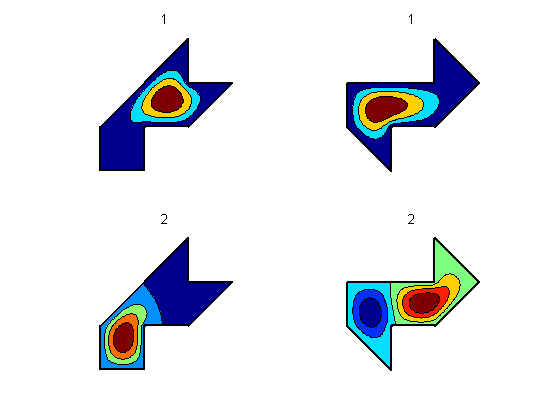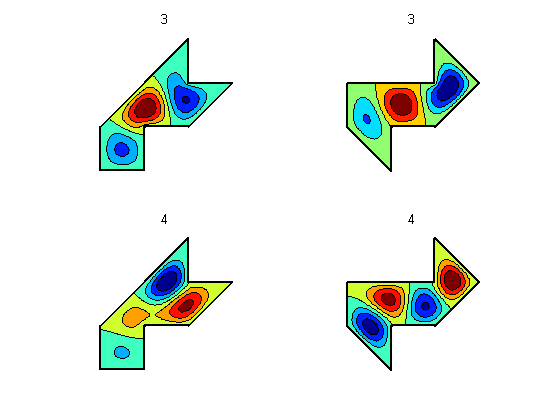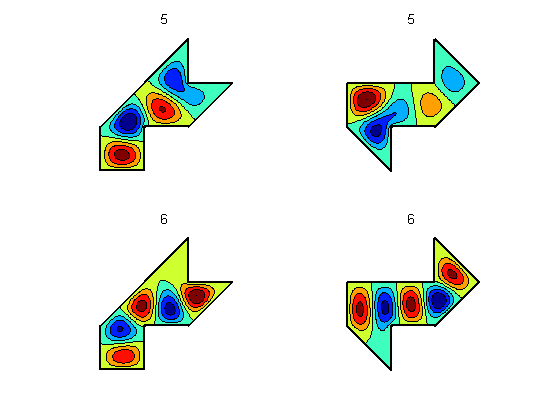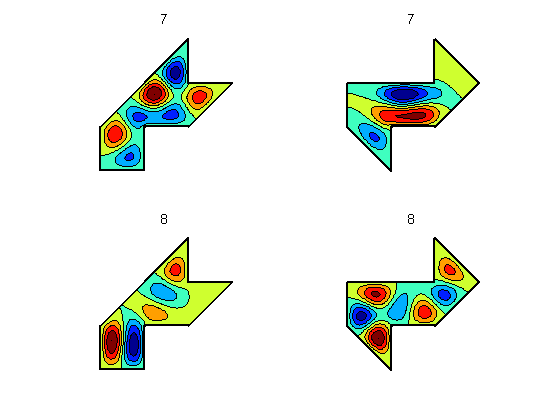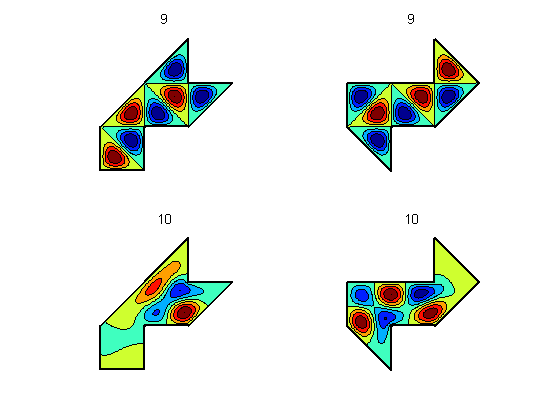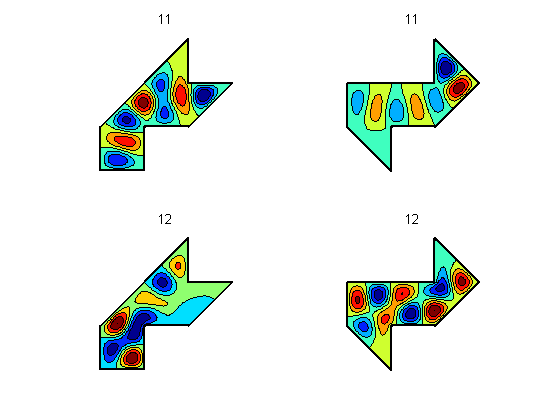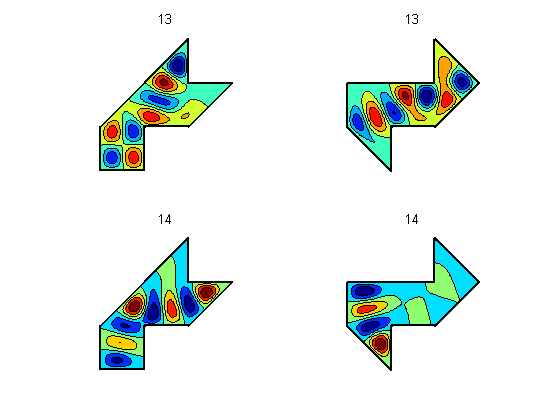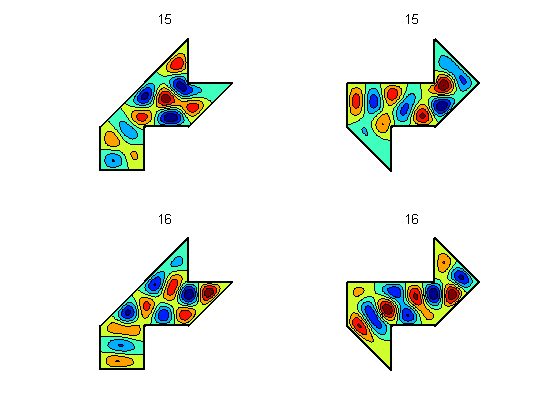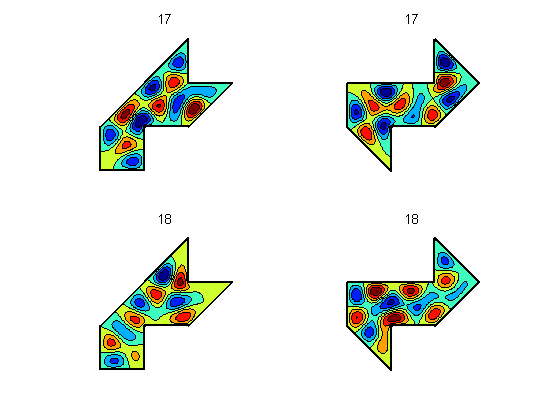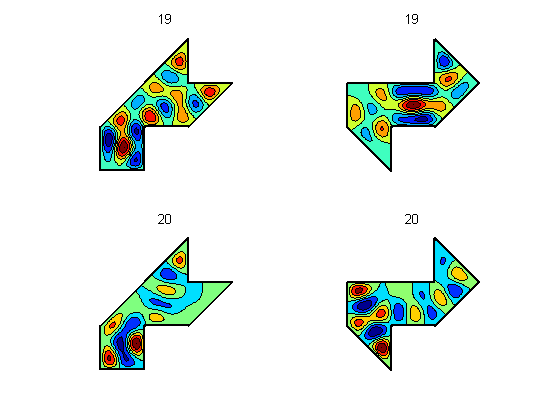#### Continuous solution for ninth eigenfunction.

The ninth eigenfunction of either region is the first eigenfunction of the isosceles triangle subregion, reflected to fill out the entire region. The corresponding eigenvalue of the continuous problem is $5 \pi^2$. $$v_9 = \sin{2 \pi x} \sin{\pi y} - \sin{\pi x} \sin{2 \pi y}$$
   v9continuous = @(x,y) sin(2*pi*x).*sin(pi*y) - sin(pi*x).*sin(2*pi*y);

figure(gcf+1)
set(gcf,'color','white')
for d = 1:2
u = v9continuous(x,flipud(y));
subplot(2,2,d)
vs = vertices{d};
[in,on] = inpolygon(x,y,vs(1,:),vs(2,:));
u(~in) = 0.5*u(~in);
s = max(abs(u(:)));
contourf(x,y,u,s*(-1:1/4:1))
line(vs(1,:),vs(2,:),'color','black','linewidth',3)
title('Continuous v9')
axis([-0.1 3.1 -0.1 3.1])
axis square off
end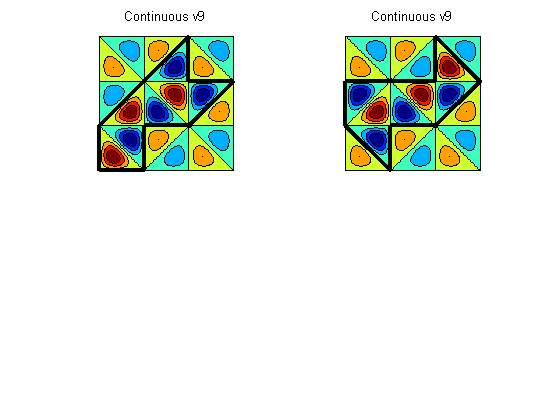#### References

Published with MATLAB® 7.14

|

### 댓글

댓글을 남기려면 링크 를 클릭하여 MathWorks 계정에 로그인하거나 계정을 새로 만드십시오.